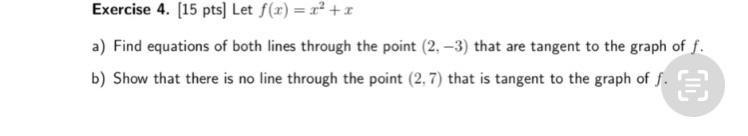Question:

# Exercise 4. (15 pts] Let f(x) = x2 + x a) Find equations of both lines through the point (2.-3) that are tangent to the graph ofExercise 4. (15 pts] Let f(x) = x2 + x a) Find equations of both lines through the point (2.-3) that are tangent to the graph of f. b) Show that there is no line through the point (2,7) that is tangent to the graph of f. &